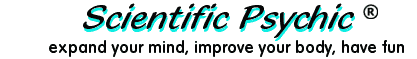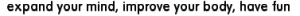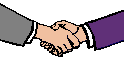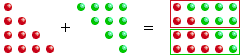Index#The Handshake Problem

At a party, everyone shook hands with everybody else. There were 66 handshakes. How many people were at the party?

Handshakes are used traditionally as greetings, but they are also used to seal an agreement when a business transaction has been mutually accepted. A handshake is sometimes used to characterize the personality of an individual. A firm handshake is interpreted to indicate an assertive person or an extroverted personality, whereas a less firm or limp handshake is viewed as a sign of weakness and lack of confidence. Some diseases, such as influenza, can be spread by shaking hands with an infected individual and then touching one's face. (See Hygiene)

With two people (A and B), there is one handshake
(A with B).

With three people (A, B, and C), there are three handshakes
(A with B and C;   B with C).

With four people (A, B, C, and D), there are six handshakes
(A with B, C, and D;   B with C and D;   C with D).

In general, with n+1 people, the number of handshakes is the sum of the first n consecutive numbers: 1+2+3+ ... + n.

Since this sum is n(n+1)/2, we need to solve the equation n(n+1)/2 = 66.

This is the quadratic equation n2+ n -132 = 0. Solving for n, we obtain 11 as the answer and deduce that there were 12 people at the party.

Since 66 is a relatively small number, you can also solve this problem with a hand calculator. Add 1 + 2 = + 3 = +... etc. until the total is 66. The last number that you entered (11) is n.

Carl Friedrich Gauss (1777-1855) is credited with finding the formula for computing the sum of the first n consecutive numbers when he was an elementary school student, at age 8. The teacher had asked the students to compute the sum (S) of the first 100 integers. To the teacher's astonishment, Gauss was able to do it very quickly by noticing that the sum of the sequence and the reverse sequence produced a series of constants.

S =   1 +  2 +  3 + ... + 100
S = 100 + 99 + 98 + ... +   1
2S = 101 +101 +101 + ... + 101 = 100×101

S = (100×101)/2 = 5,050

The symmetry of the solution can also be observed with a graphical representation: